# When Worlds Collide: Simulating Circle-Circle Collisions

Most collision detection in computer games is done using the AABB technique: very simply, if two rectangles intersect, then a collision has occurred. It's fast, efficient, and incredibly effective – for rectangular objects. But what if we wanted to smash circles together? How do we calculate the collision point, and where do the objects go after? It's not as hard as you might think...

Note: Although this tutorial is written using AS3 and Flash, you should be able to use the same techniques and concepts in almost any game development environment.

Preview image taken from this classic Psdtuts+ tutorial.

## Step 1: Create Some Balls

I'm going to gloss over this step, because if you can't create basic sprites, the rest of the tutorial is going to be a bit beyond you.

Suffice to say, we have a starting point. Below is a very rudimentary simulation of balls bouncing around a screen. If they touch the edge of the screen, they bounce back.

There is, however, one important thing to note: often, when you create sprites, the top left part is set to the origin (0, 0) and the bottom right is (width, height). Here, the circles we have created are centred on the sprite.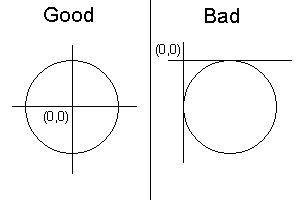This makes everything considerably easier, since if the circles are not centered, for more or less every calculation we have to offset it by the radius, perform the calculation, then reset it back.

You can find the code up to this point in the v1 folder of the source download.

## Step 2: Check for Overlaps

The first thing we want to do is check if our balls are near each other. There are a few ways to do this, but because we want to be good little programmers, we're going to start off with an AABB check.

AABB stands for axis-aligned bounding box, and refers to a rectangle drawn to fit tightly around an object, aligned so that its sides are parallel to the axes.

An AABB collision check does not check whether the circles overlap each other, but it does let us know if they are near each other. Since our game only uses four objects, this is not necessary, but if we were running a simulation with 10,000 objects, then doing this little optimisation would save us many CPU cycles.

So here we go:

This should be fairly straightforward: we're establishing bounding boxes the size of each ball's diameter squared.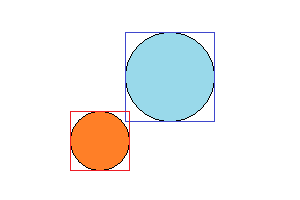Here, a “collision” has occurred - or rather, the two AABBs overlap, which means the circles are close to each other, and potentially colliding.

Once we know the balls are in proximity, we can be a little bit more complex. Using trigonometery, we can determine the distance between the two points:

Here, we're using Pythagoras' theorem, a^2 + b^2 = c^2, to figure out the distance between the two circles' centers.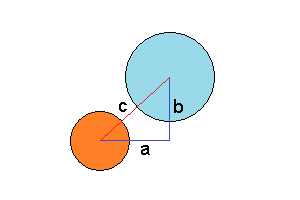We don't immediately know the lengths of a and b, but we do know the co-ordinates of each ball, so it's trivial to work out:

We then solve for c with a bit of algebraic rearrangement: c = Math.sqrt(a^2 + b^2) - hence this part of the code:

We then check this value against the sum of the radii of the two circles:

Why do we check against the circles' combined radii? Well, if we look at the picture below, then we can see that – no matter at what angle the circles are touching - if they are touching the line then c is equal to r1 + r2.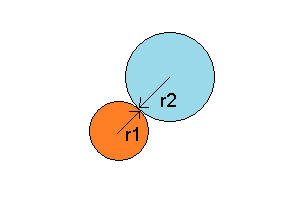So – if c is equal to or less than r1 + r2, then the circles have to be touching. Simple!

Note as well that, in order to calculate collisions properly, you will likely want to move all your objects first, and then perform collision detection on them. Otherwise you may have a situation where Ball1 updates, checks for collisions, collides, then Ball2 updates, is no longer in the same area as Ball1, and reports no collision. Or, in code terms:

is much better than

You can find the code up to this point in the v2 folder of the source download.

## Step 3: Calculate Collision Points

This part isn't really required for ball collisions, but it's pretty cool, so I'm throwing it in. If you just want to get everything bouncing around, feel free to skip to the next step.

It can sometimes be handy to work out the point at which two balls have collided. If you want to, for example, add a particle effect (perhaps a little explosion), or you're creating some sort of guideline for a snooker game, then it can be useful to know the collision point.

There are two ways to work out this out: the right way, and the wrong way.

The wrong way, which many tutorials use, is to average the two points:

This works, but only if the balls are same size.

The formula we want to use is slightly more complicated, but works for balls of all sizes:

This uses the radii of the balls to give us the true x and y co-ordinates of the collision point, represented by the blue dot in the demo below.

You can find the code up to this point in the v3 folder of the source download.

## Step 4: Bouncing Apart

Now, we know when our objects collide into each other, and we know their velocity and their x and y locations. How do we work out where they travel next?

We can do something called elastic collision.

Trying to explain in words how an elastic collision works can be complicated - the following animated image should make things clearer.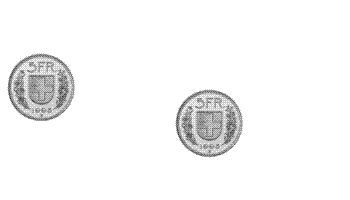Image from http://en.wikipedia.org/wiki/Elastic_collision

Simply, we're using more triangles.

Now, we can work out the direction each ball takes, but there may be other factors at work. Spin, friction, the material the balls are made from, mass and countless other factors can be applied trying to make the “perfect” collision. We're going to only worry about one of these: mass.

In our example, we're going to assume that the radius of the balls we're using is also their mass. If we were striving for realism, then this would be inaccurate since – assuming the balls were all made from the same material – the mass of the balls would be proportional to either their area or volume, depending on whether or not you wanted to consider them discs or spheres. However, since this is a simple game, using their radii will suffice.

We can use the following formula to calculate the change in x velocity of the first ball:

So, let's go over this simply:

• Assume both balls have the same mass (we'll say 10).
• The first ball is moving at 5 units / update (to the right). The second ball is moving -1 units/update (to the left).

In this case, assuming a head-on collision, the first ball will start moving at -1 unit/update. (To the left). Because the balls' masses are equal, the collision is direct and no energy has been lost, they balls will have “traded” speeds. Changing any of these factors will obviously change the outcome.

(If you use the same calculation to figure out the second ball's new speed, you'll find that it's moving at 5 units/update, to the right).

We can use this same formula to calculate the x/y velocities of both balls after the collision:

Hopefully it's evident that each calculation is the same, simply replacing the values accordingly.

Once this is done, we have the new velocities of each ball. So are we done? Not quite.

Earlier, we made sure to do all our position updates at once, and then check for collisions. This means that when we check for colliding balls, its very likely that one ball will be “in” another – so when the collision detection is called, both the first ball and the second ball will register that collision, meaning that our objects may get stuck together.

(If the balls are heading together, the first collision will reverse their directions – so they move apart – and the second collision will reverse their direction again, causing them to move together).

There are several ways to deal with this, such as implementing a Boolean which checks whether the balls have already collided this frame, but the easiest way is simply to move each ball by the new velocity. This means, in principle, that the balls should move apart by the same amount of speed that they moved together – placing them a distance apart equal to the frame before they collided.

And that's it!

You can see the final product here:

And source code up to this part is available in the v4 folder of the source download.